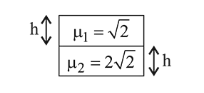# A vessel of depth 2 h is half filled with a liquidQuestion:

A vessel of depth $2 \mathrm{~h}$ is half filled with a liquid of refractive index $2 \sqrt{2}$ and the upper half with another liquid of refractive index $\sqrt{2}$. The liquids are immiscible. The apparent depth of the inner surface of the bottom of vessel will be:

1. $\frac{h}{\sqrt{2}}$

2. $\frac{h}{2(\sqrt{2}+1)}$

3. $\frac{h}{3 \sqrt{2}}$

4. $\frac{3}{4} h \sqrt{2}$

Correct Option: 4

Solution:

(4) Apparent depth,$D=\frac{t_{1}}{\mu_{1}}+\frac{t_{2}}{\mu_{2}}=\frac{h}{\sqrt{2}}+\frac{h}{2 \sqrt{2}}=\frac{3 h}{2 \sqrt{2}}=\frac{3 h \sqrt{2}}{4}$